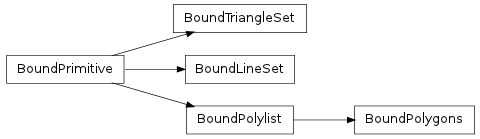#### Next topic

A line set bound to a transform matrix and materials mapping.

• If bs is an instance of collada.lineset.BoundLineSet, len(bs) returns the number of lines in the set and bs[i] returns the ith line in the set.__init__(ls, matrix, materialnodebysymbol)

Create a bound line set from a line set, transform and material mapping. This gets created when a line set is instantiated in a scene. Do not create this manually.

Methods

 __init__(ls, matrix, materialnodebysymbol) Create a bound line set from a line set, transform and material mapping. lines() Iterate through all the lines contained in the set. shapes() Iterate through all the lines contained in the set.

Attributes

 normal Read-only numpy.array of size Nx3 where N is the number of normal values in the normal_index Read-only numpy.array of size Nx3 where N is the number of vertices in the primitive. texcoord_indexset Read-only tuple of texture coordinate index arrays. texcoordset Read-only tuple of texture coordinate arrays. vertex Read-only numpy.array of size Nx3 where N is the number of vertex points in the vertex_index Read-only numpy.array of size Nx3 where N is the number of vertices in the primitive.
normal

Read-only numpy.array of size Nx3 where N is the number of normal values in the primitive’s normal source array. The values will be transformed according to the bound transformation matrix.

normal_index

Read-only numpy.array of size Nx3 where N is the number of vertices in the primitive. To get the actual normal values, one can use this array to select into the normals array, e.g. normal[normal_index]. The values will be transformed according to the bound transformation matrix.

texcoord_indexset

Read-only tuple of texture coordinate index arrays. Each value is a numpy.array of size Nx2 where N is the number of vertices in the primitive. To get the actual texture coordinates, one can use the array to select into the texcoordset array, e.g. texcoordset[texcoord_indexset] would select the first set of texture coordinates. The values will be transformed according to the bound transformation matrix.

texcoordset

Read-only tuple of texture coordinate arrays. Each value is a numpy.array of size Nx2 where N is the number of texture coordinates in the primitive’s source array. The values will be transformed according to the bound transformation matrix.

vertex

Read-only numpy.array of size Nx3 where N is the number of vertex points in the primitive’s vertex source array. The values will be transformed according to the bound transformation matrix.

vertex_index

Read-only numpy.array of size Nx3 where N is the number of vertices in the primitive. To get the actual vertex points, one can use this array to select into the vertex array, e.g. vertex[vertex_index]. The values will be transformed according to the bound transformation matrix.

lines()

Iterate through all the lines contained in the set.